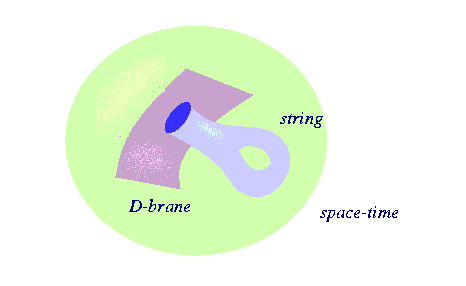P-branes ---Brane theory?

There are higher dimensional objects in string theory with dimensions from zero (points) to nine, called p-branes. In terms of branes, what we usually call a membrane would be a two-brane, a string is called a one-brane and a point is called a zero-brane.

As we have seen the equations require boundary conditions

A special class of branes is found when the
Dirichlet boundary conditions are used so  the endpoint is fixed to move only on some                                     manifold  D-branes are the result of thisD-branes are actually dynamical objects which have fluctuations and can move around. For example they interact with gravity. In the diagram below we see one way in which an closed string (graviton) can interact with a D2-brane. Notice how the closed string becomes an open string with endpoints on the D-brane at the intermediate point in the interaction.not just strings

D-branes and Black holes